• 最小均方误差最小二乘有什么区别？ https://www.zhihu.com/question/27200164/answer/62411596
最小均方误差和最小二乘有什么区别？

https://www.zhihu.com/question/27200164/answer/62411596

展开全文• 利用最小二乘法可以简便地求得未知的数据，并使得这些求得的数据实际数据之间误差的平方和为最小。最小二乘法还可用于曲线拟合。其他一些优化问题也可通过最小化能量或最大化熵用最小二乘法来表达。 以最简单的...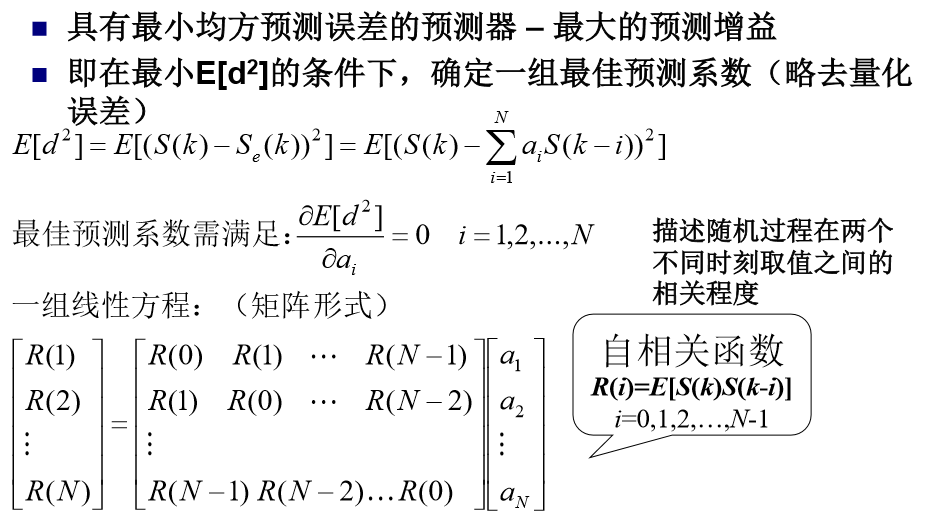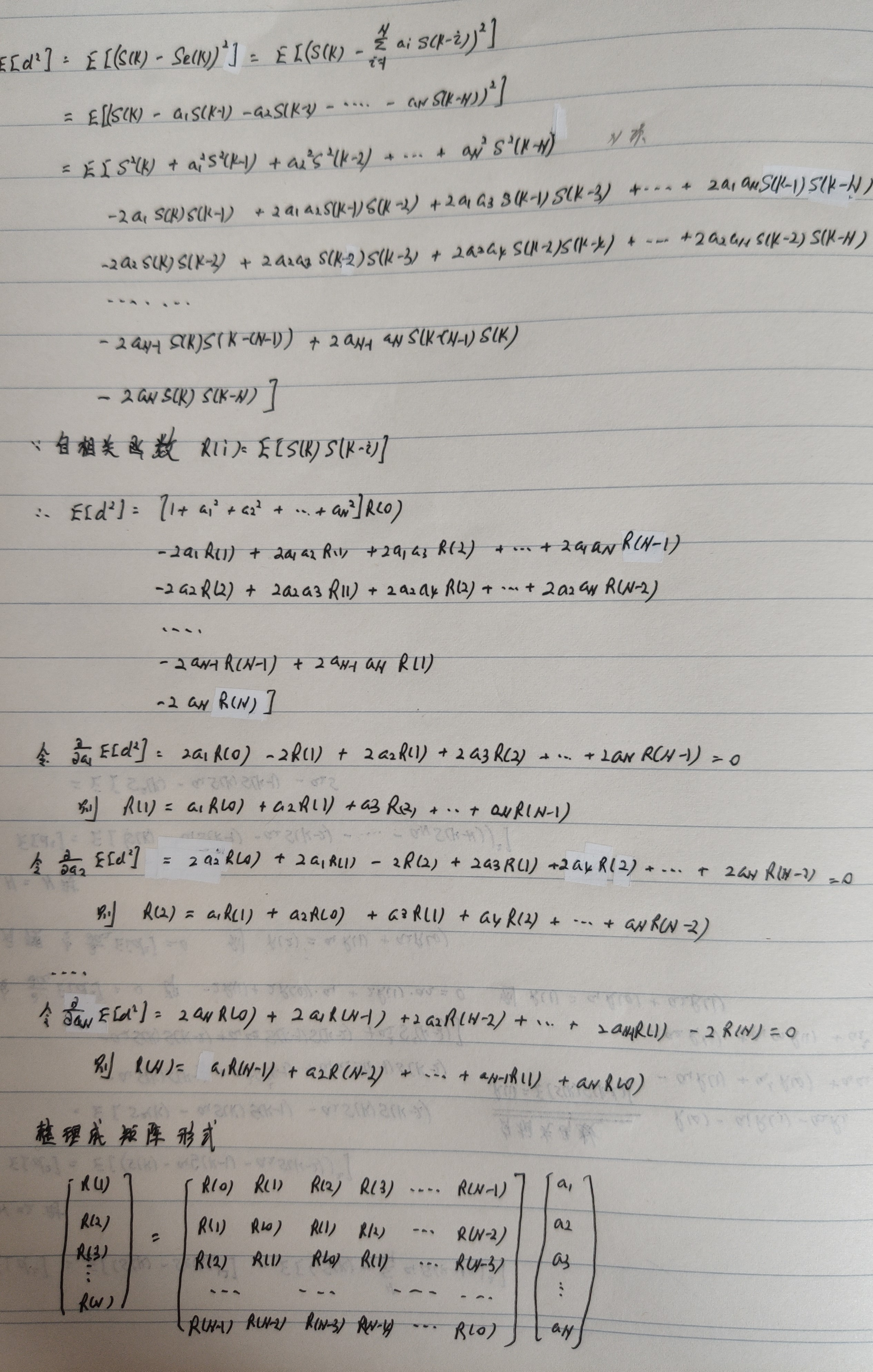最小二乘法（又称最小平方法）是一种数学优化技术。它通过最小化误差的平方和寻找数据的最佳函数匹配。利用最小二乘法可以简便地求得未知的数据，并使得这些求得的数据与实际数据之间误差的平方和为最小。最小二乘法还可用于曲线拟合。其他一些优化问题也可通过最小化能量或最大化熵用最小二乘法来表达。
基本公式
考虑超定方程组（超定指方程个数大于未知量个数）：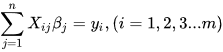其中m代表有m个等式，n代表有n个未知数β，m>n ；将其进行向量化后为：Xβ=y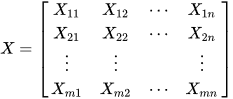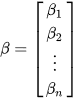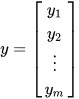显然该方程组一般而言没有解，所以为了选取最合适的β让该等式"尽量成立"，引入残差平方和函数S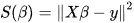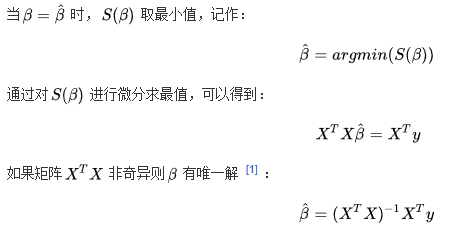方法
以最简单的一元线性模型来解释最小二乘法。什么是一元线性模型呢？监督学习中，如果预测的变量是离散的，我们称其为分类（如决策树，支持向量机等），如果预测的变量是连续的，我们称其为回归。回归分析中，如果只包括一个自变量和一个因变量，且二者的关系可用一条直线近似表示，这种回归分析称为一元线性回归分析。如果回归分析中包括两个或两个以上的自变量，且因变量和自变量之间是线性关系，则称为多元线性回归分析。对于二维空间线性是一条直线；对于三维空间线性是一个平面，对于多维空间线性是一个超平面。
对于一元线性回归模型, 假设从总体中获取了n组观察值（X1，Y1），（X2，Y2）， …，（Xn，Yn）。对于平面中的这n个点，可以使用无数条曲线来拟合。要求样本回归函数尽可能好地拟合这组值。综合起来看，这条直线处于样本数据的中心位置最合理。 选择最佳拟合曲线的标准可以确定为：使总的拟合误差（即总残差）达到最小。有以下三个标准可以选择：
（1）用“残差和最小”确定直线位置是一个途径。但很快发现计算“残差和”存在相互抵消的问题。
（2）用“残差绝对值和最小”确定直线位置也是一个途径。但绝对值的计算比较麻烦。
（3）最小二乘法的原则是以“残差平方和最小”确定直线位置。用最小二乘法除了计算比较方便外，得到的估计量还具有优良特性。这种方法对异常值非常敏感。
最常用的是普通最小二乘法（ Ordinary Least Square，OLS）：所选择的回归模型应该使所有观察值的残差平方和达到最小。（Q为残差平方和）- 即采用平方损失函数。
样本回归模型：（其中ei为样本（Xi,Yi）的误差）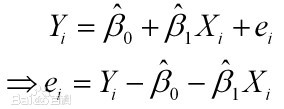平方损失函数：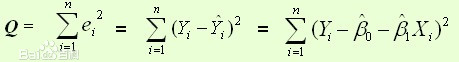则通过Q最小确定这条直线，即确定β0和β1，把它们看作是Q的函数，就变成了一个求极值的问题，可以通过求导数得到。求Q对两个待估参数的偏导数：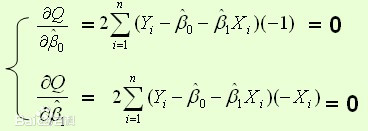根据数学知识我们知道，函数的极值点为偏导为0的点。
解得：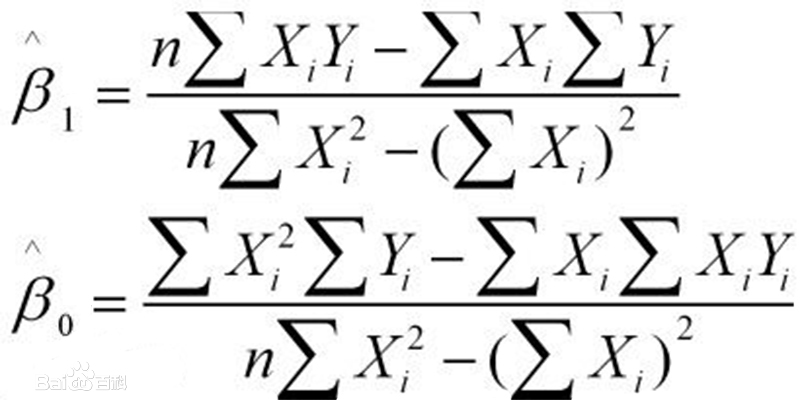这就是最小二乘法的解法，就是求得平方损失函数的极值点。
梯度下降法（英语：Gradient descent）是一个一阶最优化算法。 要使用梯度下降法找到一个函数的局部极小值，必须向函数上当前点对应梯度（或者是近似梯度）的反方向的规定步长距离点进行迭代搜索。
我们给出线性回归的损失函数，为了方便，不带正则项：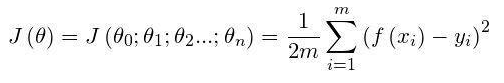其中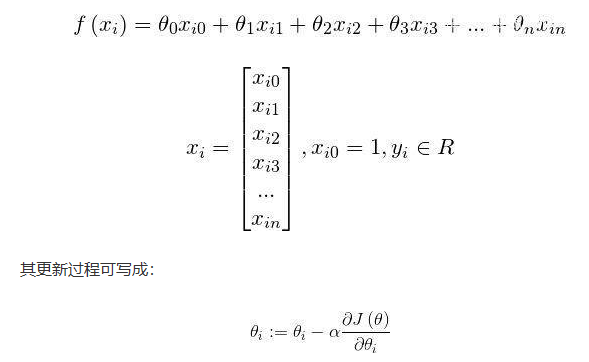具体的梯度下降流程：
第一步：先随便假设一组θ,你要是喜欢可以全部取0
第二步循环迭代:
第一次迭代：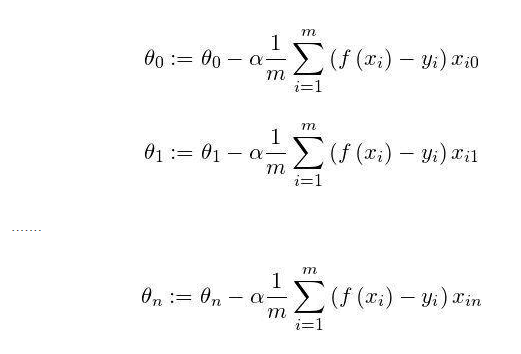第二次迭代：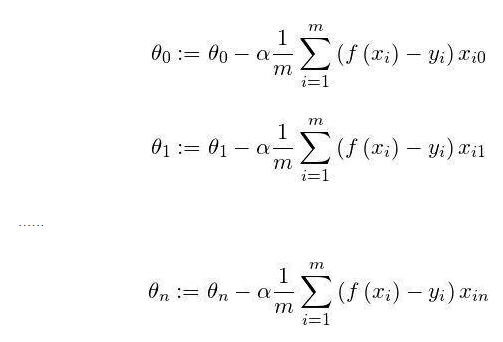…
第x次迭代：…
第三步，满足要求，循环结束，得到θ
最小二乘法跟梯度下降法都是通过求导来求损失函数的最小值，那它们有什么异同？
相同：
1.本质相同：两种方法都是在给定已知数据（independent & dependent variables）的前提下对dependent variables算出出一个一般性的估值函数。然后对给定新数据的dependent variables进行估算。
2.目标相同：都是在已知数据的框架内，使得估算值与实际值的总平方差尽量更小（事实上未必一定要使用平方），估算值与实际值的总平方差的公式为：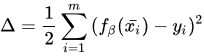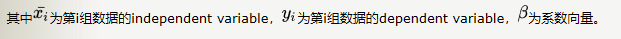不同：
1.实现方法和结果不同：最小二乘法是直接对Δ求导找出全局最小，是非迭代法。而梯度下降法是一种迭代法，先给定一个β，然后向Δ下降最快的方向调整β，在若干次迭代之后找到局部最小。梯度下降法的缺点是到最小点的时候收敛速度变慢，并且对初始点的选择极为敏感，其改进大多是在这两方面下功夫。
牛顿法
牛顿法是一种在实数域和复数域上近似求解方程的方法。方法使用函数f (x)的泰勒级数的前面几项来寻找方程f(x)=0的根。牛顿法最大的特点就在于它的收敛速度很快。
用牛顿迭代法解非线性方程，是把非线性方程f(x)=0线性化的一种近似方法。把f(x)在点x0的某邻域内展开成泰勒级数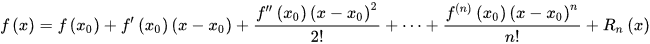取其线性部分（即泰勒展开的前两项），并令其等于0，即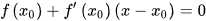以此作为非线性方程f(x)=0的近似方程，若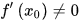则其解为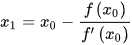得到牛顿迭代法的一个迭代关系式：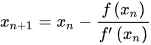已经证明，如果是连续的，并且待求的零点是孤立的，那么在零点周围存在一个区域，只要初始值位于这个邻近区域内，那么牛顿法必定收敛。 并且，如果不为0, 那么牛顿法将具有平方收敛的性能. 粗略的说，这意味着每迭代一次，牛顿法结果的有效数字将增加一倍。
高斯牛顿法
高斯一牛顿迭代法(Gauss-Newton iteration method)是非线性回归模型中求回归参数进行最小二乘的一种迭代方法，该法使用泰勒级数展开式去近似地代替非线性回归模型，然后通过多次迭代，多次修正回归系数，使回归系数不断逼近非线性回归模型的最佳回归系数，最后使原模型的残差平方和达到最小。其直观思想是先选取一个参数向量的参数值β，若函数ft(Xt，β)在β0附近有连续二阶偏导数，则在β0的邻域内可近似地将ft(Xt，β)看作是线性，因而可近似地用线性最小二乘法求解
高斯-牛顿法的一般步骤如下所示
(1) 初始值的选择。其方法有三种：
一是根据以往的经验选定初始值；
二是用分段法求出初始值；
三是对于可线性化的非线性回归模型，通过线性变换，然后施行最小平方法求出初始值
(2)泰勒级数展开式。设非线性回归模型为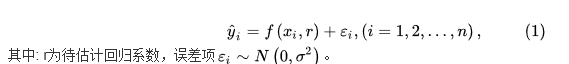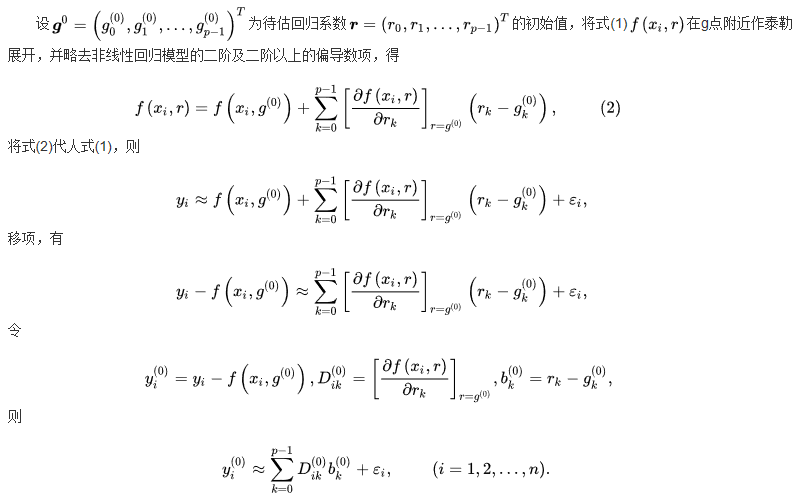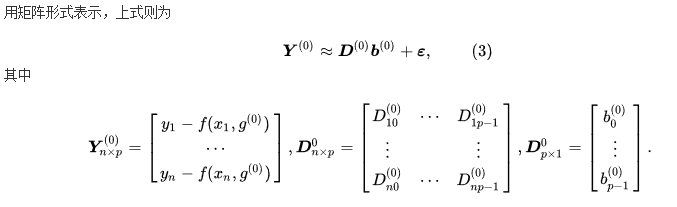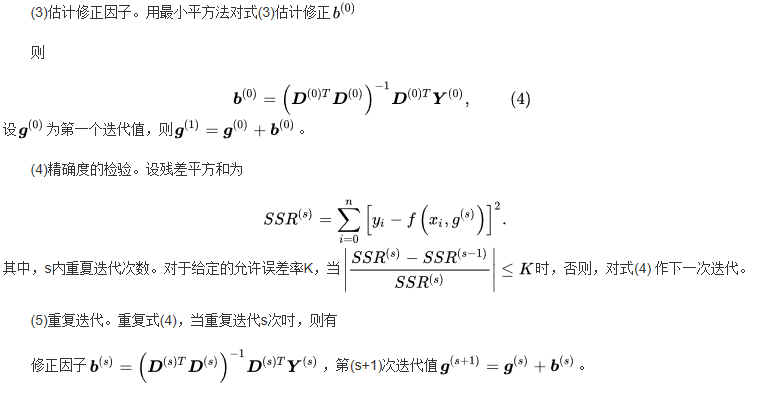最小二乘法C++实现
/*
最小二乘法C++实现
参数1为输入文件
输入 ： x
输出： 预测的y
*/
#include<iostream>
#include<fstream>
#include<vector>
using namespace std;
class LeastSquare{
double a, b;
public:
LeastSquare(const vector<double>& x, const vector<double>& y)
{
double t1=0, t2=0, t3=0, t4=0;
for(int i=0; i<x.size(); ++i)
{
t1 += x[i]*x[i];
t2 += x[i];
t3 += x[i]*y[i];
t4 += y[i];
}
a = (t3*x.size() - t2*t4) / (t1*x.size() - t2*t2);  // 求得β1
b = (t1*t4 - t2*t3) / (t1*x.size() - t2*t2);        // 求得β2
}
double getY(const double x) const
{
return a*x + b;
}
void print() const
{
cout<<"y = "<<a<<"x + "<<b<<"\n";
}
};
int main(int argc, char *argv[])
{
if(argc != 2)
{
cout<<"Usage: DataFile.txt"<<endl;
return -1;
}
else
{
vector<double> x;
ifstream in(argv);
for(double d; in>>d; )
x.push_back(d);
int sz = x.size();
vector<double> y(x.begin()+sz/2, x.end());
x.resize(sz/2);
LeastSquare ls(x, y);
ls.print();
cout<<"Input x:\n";
double x0;
while(cin>>x0)
{
cout<<"y = "<<ls.getY(x0)<<endl;
cout<<"Input x:\n";
}
}
}
参考：
百度百科
https://www.cnblogs.com/iamccme/archive/2013/05/15/3080737.html
https://baijiahao.baidu.com/s?id=1639202882632470513&wfr=spider&for=pc


展开全文• 最小二乘法 在构建线性回归模型时，我们通过最小二乘法获得均方误差（损失函数），我们需要得到均方误差的最小值，从而真实值假设值的差异也是最小的，所以可以得到最合适的回归系数W。 ...
最小二乘法
在构建线性回归模型时，我们通过最小二乘法获得均方误差（损失函数），我们需要得到均方误差的最小值，从而真实值与假设值的差异也是最小的，所以可以得到最合适的回归系数W。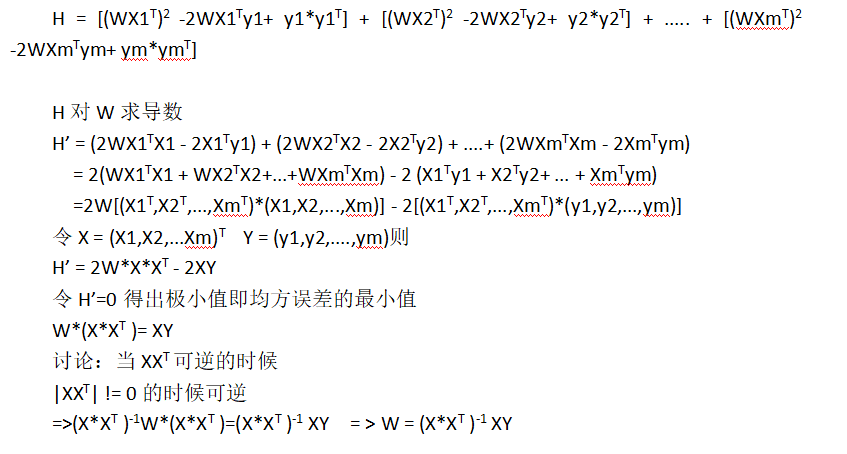当H的导数为0时得到最小值，通过我们对导数求导的计算推导可得
公式：w*(x*x^T)=XY
xx^T可逆的时候 可得最合适w
但是当xx^T不可逆的时候，m<n  w的最合适值无法求得。
这时候我们要思考解决办法。
这是我们训练出的模型可能会出现过拟合问题，泛化能力减弱。
这是我们通过根据样本的特征，用正则化岭回归或者Lasso回归解决过拟合问题
服从逻辑斯蒂分布的sigmoid函数
先带大家了解一下逻辑斯蒂分布的sigmoid函数，sigmoid函数是一种主要解决二分类问题的函数，就是要把一个回归问题通过阀值进行二分类。
sigmoid函数它是任意阶可导的凸函数，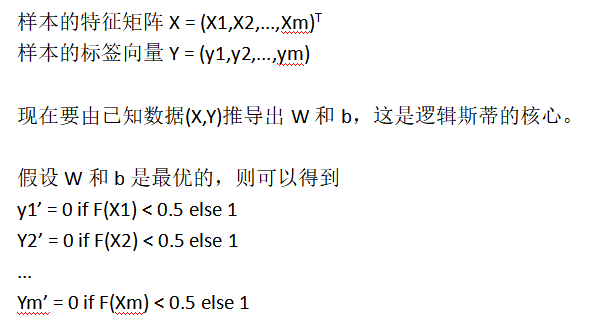sigmoid函数图像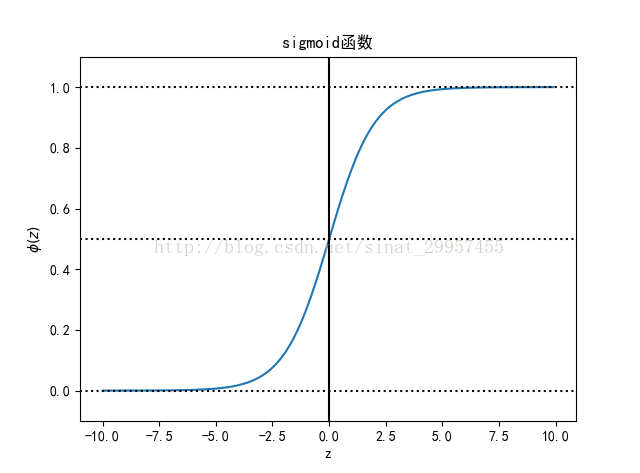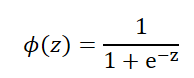逻辑斯蒂回归
逻辑斯蒂回归实际上是二分类问题，逻辑斯蒂回归是针对线性可分问题的一种易于实现而且性能优异的分类模型，是使用最为广泛的分类模型之一。逻辑斯蒂与线性回归类似，寻找一个合适的函数进行分类，这个函数就我们要找的分类函数，这个过程非常关键，我们要对我们的数据有一定了解通过数据来预测函数的大概形式，是使用线性函数还是非线性函数。
然后构造损失函数，损失函数是预测输出与训练特征的与真实数据的偏差，我们要保证二者的偏差保持最小，以求得最优得模型。
假设某件事发生的概率为p，那么这件事不发生的概率为(1-p)，我们称p/(1-p)为这件事情发生的几率。取这件事情发生几率的对数，定义为logit§，所以logit§为。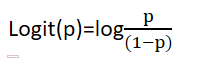最大似然估计
在线性方程中
因为逻辑斯蒂函数中输出的值保持0和1所以我们用最小二乘法的方法求出w和b方法明显无法得到我们想要的目标值，这个时候我们选择最大似然估计来解决这个问题，
最大似然估计将事件变成概率事件来解决。
最大似然估计推导的一个概率问题，即X1对应y1这件事发生的概率P1，特征X2对应标签y2这件事发生的概率为P2，…，Xm对应标签ym这件事的概率Pm;
则所有的特征和标签都对应发生的总概率是：P = P1p2…Pm
最大似然估计求的求是P的最大值
现在问题关键是P取最大值的时候W的取值情况,，这个过程就是极大似然估计。
1.构建损失函数（损失函数）
F(Z) = 1/(1+e^(-z)) =>F(x) = 1/(1+e(-W*XT - b))
似然函数,即Xi对应yi的概率
P(Xi,Yi) = [F(Xi)Yi]*[(1-F(Xi))(1-Yi)]
将所有的样本带入
P(X1,Y1) = [F(X1)Y1]*[(1-F(X1))(1-Y1)]
P(X2,Y2) = [F(X2)Y2]*[(1-F(X2))(1-Y2)]
…
P(Xm,Ym) = [F(Xm)Ym]*[(1-F(Xm))(1-Ym)]
这m个是相互独立事件，则他们同时发生的概率是：
L(w,b) = P(X1,Y1)P(X2,Y2)…P(Xm,Ym)
= [F(X1)Y1]*[(1-F(X1))(1-Y1)][F(X2)Y2]*[(1-F(X2))(1-Y2)]…*[F(Xm)Ym]*[(1-F(Xm))(1-Ym)] ，这个函数就是这个数据模型的似然函数（也是机器学习的损失函数）
2.求似然函数L(w,b)的最大值
如果对L(W,b)求导或者求偏导计算过程非常复杂
例如：(wv)’ = w’v + wv’   (wvp)’ = w’vp+wv’p + wvp’
两边同时取自然对数
l(w,b)=lnL(W,b)=Ln{[F(X1)Y1]*[(1-F(X1))(1-Y1)][F(X2)Y2]*[(1-F(X2))(1-Y2)]…[F(Xm)Ym]*[(1-F(Xm))(1-Ym)] } = ln[F(X1)Y1]*[(1-F(X1))(1-Y1)] + ln[F(X2)Y2]*[(1-F(X2))(1-Y2)] + …+[F(Xm)Ym]*[(1-F(Xm))(1-Ym)] = [Y1lnF(X1) +Y2lnF(X2) + …+YmlnF(Xm) ] + [(1-Y1)*ln(1-F(X1)) + (1-Y2)*ln(1-F(X2))+…+(1-Ym)*ln(1-F(Xm))]
方案一：求导
l(W,b)’ = 0
就可以得到W的极大似然估计值，这个极大似然估计值就是最大似然估计值
方案二：梯度下降


展开全文• 文章目录预测误差均方值推导及最小二乘法解法总结一、预测误差均方值最小值推导二、最小二乘法总结1、最小二乘法2、梯度下降法（1）原理（2）算法过程（3）存在问题3、牛顿法（1）原理梯度下降法比较：（2）算法...
文章目录预测误差均方值推导及最小二乘法解法总结一、预测误差均方值最小值推导二、最小二乘法总结1、最小二乘法2、梯度下降法（1）原理（2）算法过程（3）存在问题3、牛顿法（1）原理（2）算法过程（3）存在问题4、高斯牛顿法
预测误差均方值推导及最小二乘法解法总结
一、预测误差均方值最小值推导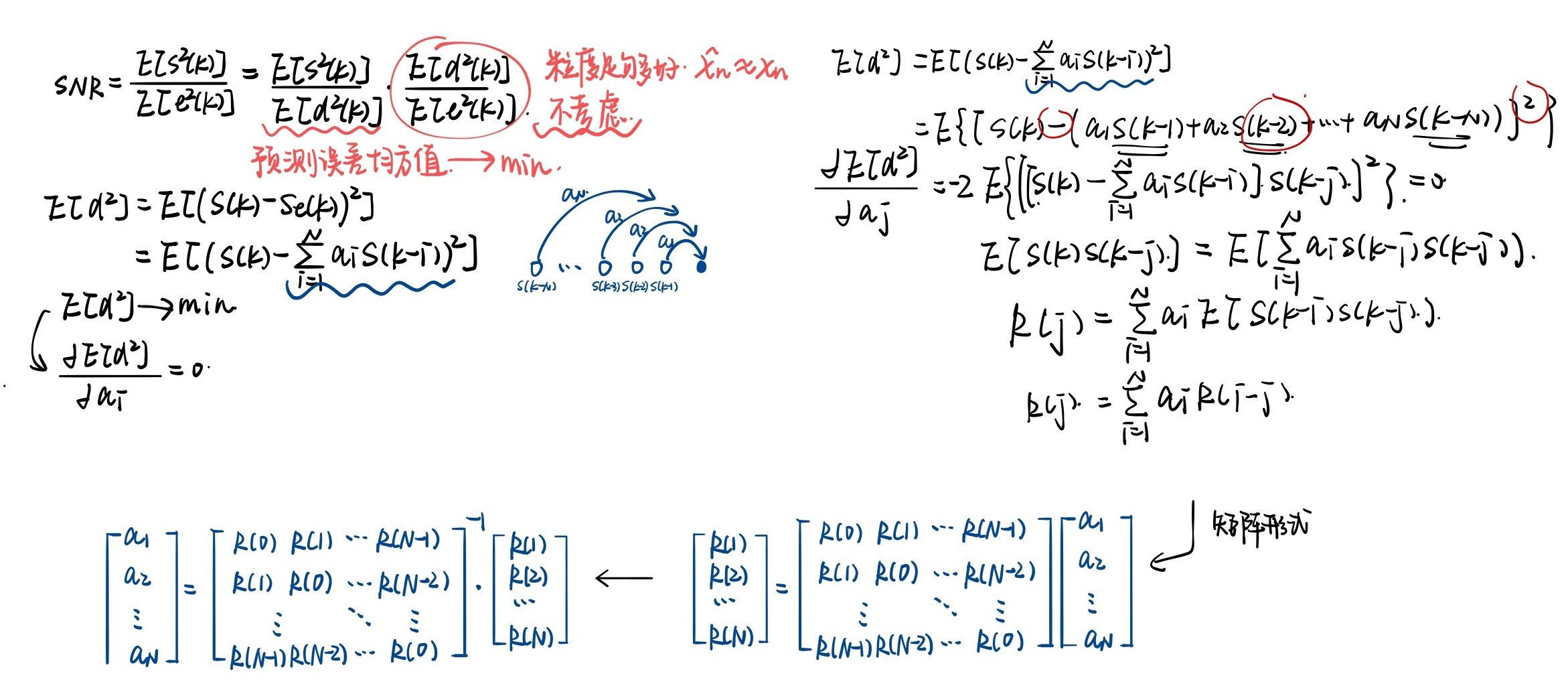二、最小二乘法总结
1、最小二乘法
最小二乘法（英语：least squares method），又称最小平方法，是一种数学优化方法。它通过最小化误差的平方和寻找数据的最佳函数匹配。
利用最小二乘法可以简便的求得未知的数据，并使得求得的数据与实际数据之间误差的平方和（目标函数）为最小。
目标函数形式如下；
$\text{目标函数} =\sum \left( \text{观测值} -\text{理论值} \right)^{2}$
2、梯度下降法
（1）原理
多元函数的梯度定义为：
$\nabla f\left( x\right) =\left( \frac{\partial f}{\partial x_{1}} ,\cdots ,\frac{\partial f}{\partial x_{n}} \right)^{T}$
其中∇ 称为梯度算子，它作用于一个多元函数，得到一个向量。可导函数在某一点处取得极值的必要条件是梯度为0，梯度为0的点称为函数的驻点，这是疑似极值点。需要注意的是，梯度为0只是函数取极值的必要条件而不是充分条件，即梯度为0的点可能不是极值点。
对于实际应用中的大部分函数，直接令梯度为0求得精确解不可行，因此只能转而求近似解。实现时通常采用的是迭代法，它从一个初始点x0开始，反复使用某种规则从xk移动到下一个点xk+1，构造这样一个数列，直到收敛到梯度为0的点处。即有下面的极限成立：
$\lim_{k\rightarrow +\infty } \nabla f\left( x_{k}\right) =0$
方法的核心是得到这样的由上一个点确定下一个点的迭代公式：
$x_{k+1}=h\left( x_{k}\right)$
梯度的方向是函数在给定点上升最快的方向，那么梯度的反方向就是函数在给定点下降最快的方向。故在梯度下降法中，从起始点开始，沿着函数下降最快方向以一定步长前进，经过多次迭代最终可到达梯度为0位置，此时认为已经达到极值点。
迭代公式为：
$x_{k+1}=x_{k}-\alpha f\left( x_{k}\right)$
其中α为一个接近于0的正数，称为步长（学习率），由人工设定。
（2）算法过程

确定当前位置的损失函数的梯度；

用步长乘以损失函数的梯度，得到当前位置下降的距离；

确定是否所有的𝜃𝑖,梯度下降的距离都小于𝜀，如果小于𝜀则算法终止，当前所有的𝜃𝑖(i=0,1,…n)即为最终结果。否则进入步骤4；

更新所有的𝜃，更新完毕后继续转入步骤1；

（3）存在问题

局部极小值：有些函数可能有多个局部极小值点。假设A、B、C均为函数的极小值，而只有C是函数的全局极小值，梯度下降法可能迭代到B或者C点处就终止。

​	鞍点：鞍点是指梯度为0，Hessian矩阵既不是正定也不是负定，即不定的点。在鞍点处，梯度下降法遇到了鞍点，认为已经找到了极值点，从而终止迭代过程，而这根本不是极值点。例子如下图：3、牛顿法
和梯度下降法一样，牛顿法也是寻找导数为0的点，同样是一种迭代法。核心思想是在某点处用二次函数来近似目标函数，得到导数为0的方程，求解该方程，得到下一个迭代点。因为是用二次函数近似，因此可能会有误差，需要反复这样迭代，直到到达导数为0的点处。
（1）原理
根据多元函数的泰勒展开公式，我们对目标函数在x0点处做泰勒展开，有：
$f\left( x\right) =f\left( x_{0}\right) +\nabla f\left( x_{0}\right) \left( x-x_{0}\right) +\frac{1}{2} \left( x-x_{0}\right)^{T} \nabla^{2} f\left( x_{0}\right) +o\left( \left( x-x_{0}\right)^{2} \right)$忽略二次及以上的项，并对上式两边同时求梯度，得到函数的导数（梯度向量）为：
$\nabla \left( x\right) =\nabla f\left( x_{0}\right) +\nabla^{2} f\left( x_{0}\right) f\left( x-x_{0}\right)$其中 $\nabla^{2} f\left( x_{0}\right)$ 即为Hessian矩阵，在后面我们写成H。令函数的梯度为0，则有：
$x=x_{0}-H^{-1}\nabla f\left( x_{0}\right)$从初始点x0处开始，反复计算函数在处的Hessian矩阵和梯度向量，然后用下述公式进行迭代：
$x_{k+1}=x_{k}-H^{-1}_{k}\nabla f\left( x_{k}\right)$最终会到达函数的驻点处。迭代终止的条件是梯度的模接近于0，或者函数值下降小于指定阈值。

Hessian 矩阵，是一个多元函数的二阶偏导数构成的方阵，描述了函数的局部曲率。
Hessian 矩阵就是梯度的雅可比矩阵。
假设有一实值函数f(x1,x2, … ,xn)，如果f的所有二阶偏导数都存在并在定义域内连续，那么函数f的Hessian 矩阵为：与梯度下降法比较：
和梯度下降法相比牛顿法有更快的收敛速度，但每一步迭代的成本也更高。在每次迭代中，除了要计算梯度向量还要计算Hessian矩阵，并求解Hessian矩阵的逆矩阵。

梯度法自起始点出发，在局部进行下降步步逼近极值，行进路线往往呈之字形。

而牛顿法在二阶导数的作用下，考虑函数凹凸性，直接搜索如何达到极值。在选择行进方向上，不仅考虑当前坡度是否够大，还考虑前进后坡度是否会变得更大。

综上，牛顿法比梯度下降法收敛速度更快。
（2）算法过程

给定初始值x0和精度阈值ε，设置k = 0
计算梯度gk和矩阵Hk
如果在此点处梯度的值接近于0，则达到极值点处，停止迭代
计算搜索方向 dk=-Hk-1gk
计算新的迭代点 xk=xk+1+γdk
令k = k + 1，返回步骤2

其中γ是一个人工设定的接近于0的常数，和梯度下降法一样，需要这个参数的原因是保证xk+1在xk的邻域内，从而可以忽略泰勒展开的高次项。
（3）存在问题

局部极小:与梯度下降法类似
Hessian矩阵可能不可逆
牛顿法在每次迭代时序列xi可能不会收敛到一个最优解，它甚至不能保证函数值会按照这个序列递减。解决第一个问题可以通过调整牛顿方向的步长来实现

4、高斯牛顿法
高斯牛顿法是对牛顿法的一种改进，它采用雅可比矩阵的乘积近似代替牛顿法中的二阶Hessian矩阵，从而省略了求二阶Hessian矩阵的计算。
Hessian矩阵中各元素的表达式如下：
$\frac{\partial^{2} S}{\partial \beta_{k} \partial \beta_{j} } =2\sum^{m}_{i=1} \left( \frac{\partial r_{i}}{\partial \beta_{k} } \frac{\partial r_{i}}{\partial \beta_{j} } +r_{i}\frac{\partial^{2} r_{i}}{\partial \beta_{k} \partial \beta_{j} } \right)$其中，求和号内前半部分为雅可比矩阵的元素相乘，后半部分定义为Okj。
由此，Hessian矩阵可简写为
$H=2\left( J^{T}J+O\right)$若模型拟合程度较好，则O矩阵内的ri应趋近于0，故可将后半部分忽略，直接采用雅可比矩阵的乘积近似代替二阶Hessian矩阵。
Hessian矩阵可简化为
$H=2 J^{T}J$相应的迭代公式为
$x_{k+1}=x_{k}-\left( J^{T}J\right)^{-1} J^{T}r$相比于牛顿法，计算量明显减小。
-  理解梯度下降法
-  理解牛顿法


展开全文• 最小二乘法是用来做函数拟合或求函数极值的方法，在机器...最小二乘法实质就是最小化“均方误差”，而均方误差就是残差平方和的1/m(m为样本数)，同时均方误差也是回归任务中最常用的性能度量。 1.最小二乘法的原理...
• 最小二乘准则：是一种目标：基于均方误差最小化来进行模型求解。 2) 狭义上的最小二乘法 最小二乘算法：正规方程( Normal Equation)，线性假设下的一种有闭式解的参数求解方法，最终结果为全局最优 3) 梯度下降 ...
• 最小二乘法即基于最小化均方误差求解模型的未知参数，包括线性非线性，非线性使用数值计算的迭代算法优化均方误差，例如SGD解决，线性常用于统计学中 为什么要使用均方误差度量拟合的质量？ 高斯和勒让德方假定...
• 最小二乘法是在线性回归模型最小化均方误差时使用，其实就是对误差函数求导数，然后让其等于0 ，然后解出...4、最小二乘法与正态分布 参考文献： 1、日用而不知 来看一个生活中的例子。比如说，有五把尺子： ...
• y=wi*xi+b，基于最小二乘法的线性回归：寻找参数w和b，使得w和b对x_test_data的预测值y_pred_data真实的回归目标y_test_data之间的均方误差最小。机器学习 python
• 利用最小二乘法可以简便地求得未知的数据，并使得这些求得的数据实际数据之间误差的平方和为最小。最小二乘法还可用于曲线拟合。其他一些优化问题也可通过最小化能量或最大化熵用最小二乘法来表达。 求解最小二乘...
• **1.2 最小二乘法：**基于L2范数（均方误差）最小化进行模型求解的方法。 2.计算过程 假设输入特征的值只有一个： 在线性回归中，最小二乘法就是找到一条直线，使所有样本到这条直线的距离之和最小。 求解!w和b，...机器学习 大数据 人工智能
• 最小二乘法：按均方误差（各点误差的平方和R）达到极小（即偏微分为0）构造拟合曲线的方法 设计和确定“最贴近”的拟合曲线关键要选择合适的曲线类型 例子： 二次拟合函数： 给定数据序列（xi,yi），i=
• 线性回归寻找参数w和b，使得对训练集的预测值真实的回归目标值y之间的均方误差最小均方误差是预测值真实值之差的平方和除以样本数。线性回归没有参数，这是一个优点。 import matplotlib.pyplot as plt #...机器学习
• 也称均方误差，说白了就是考察值期望（平均值）的偏离程度。 三、最小二乘法 在线程回归中： f(Xi) = WX i+ b，通过训练输入X，得到参数W和b，如何确定W和b呢？关键在于衡量f(x)y的
• 当前数据分析，机器学习比较热门，因此特别从此其基础开始学起，最小二乘回归方法，是一种常用的拟合一堆数据中因变量自变量的方法，它的优化目标是最小均方误差 ： 其matlab代码如下 n=100; N=1000; x=...Matlab
• 这些拟合的方法都是运用的了非常古老而又非常有效的方法，即最小二乘法。 今天给大家介绍一种三维球面数据的拟合方法，该方法也是运用的最小二乘的方法。旨在使拟合的半径在均方意义下误差达到最小。公式推导设拟合...
• LSMB算法比较，该算法的均方误差值（MSEv）有所降低，而权重信噪比（WSNR）和峰值信噪比（PSNR）则有一定提高，模拟实验结果验证了算法的有效性。该算法四次迭代计算以后，收敛误差降到0.20以下，具有较快的收敛...
• 提出了一种基于最小二乘法的加速度传感器误差补偿方法，用来提高列车横向加...实验结果表明，经过补偿后，加速度传感器输出信号误差明显减少，均方误差收敛到10－4。传感器的测量精度有了显著提高，完全满足工程要求。
• 最小二乘法：基于均方误差最小化来求解模型的方法，称为“最小二乘法”（least square method）。（实际上就是试图找到一条直线，使得所有样本点距离直线的“欧式距离”（Euclidean distance）之和最小） 线性回归...机器学习
• (2)当均方误差最小时，说明构造的拟合向量原始向量最为接近，这种曲线拟合的方法称为最小二乘法 (3)计算均方误差最小时的拟合系数，可以通过微积分中求解极值的方法实现   多项式拟合 (1) 多项式拟合在...
• 实验测试中分别用最小二乘法进行三次多项式、二次多项式、四次多项式的曲线拟合，并计算出了三个拟合曲线的均方误差。由运行结果可知，三次多项式的均方误差小于二次多项式的均方误差，二次多项式的均方误差小于四...
• 最小二乘法 均方误差（MSE） 平均绝对误差（MAE） 均方根误差（RMSE） R平方（ ，R ^ 2） 残差图，回归图，分布图 拦截，科夫 Đọc/ ghi文件到Google云端硬盘 培训数据测试数据 pandas-profiling
• 文章目录1. 线性回归1.1 基本形式1.2 最小二乘法推导2. Sklearn 实现参考资料 ...线性回归需按照参数 w 和 b，使得对训练集的预测值真实的回归目标值 y 之间的均方误差（MSE）最小。 均方误差（Mean...机器学习 监督学习 线性回归 Python Sklearn
• 文章目录前置知识一元线性回归...learn实现线性回归预测实战导入数据查看数据划分训练集和测试集构建和训练模型预测预测结果分析绝对误差函数均方误差函数结果分析 前置知识 【机器学习】最小二乘法（代数&矩阵推导
• Basis(基础)： MSE(Mean Square Error 均方误差)， LMS(LeastMean Square 最小均方)， LSM(Least Square Methods 最小二乘法)， MLE(MaximumLikelihood Estimation最大似然估计)， QP(Quadratic ...
• 常用机器学习数据挖掘相关术语（该... MSE(Mean Square Error 均方误差)， LMS(LeastMean Square 最小均方)， LSM(Least Square Methods 最小二乘法)， MLE(MaximumLikelihood Estimation最大似然估计)，...
• 线性回归 线性回归的目的是试图学得 衡量 f(x) y 之间的差别，均方...当均方误差的几何意义在于对应了欧氏距离，基于均方误差最小化来进行模型求解的方法称为“最小二乘法”。 对ω和b求偏导数 令①式为0得 ...
• 学习策略：最小化均方误差损失函数，求解参数w；（注意感知机的区别，此处误分类点坐标轴垂直） 求解方法： 最小二乘法，梯度下降法（两者的区别） 最小二乘法：对目标损失函数求导，导数为零的点对应的...